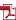# Disclosure Materials

The following are translations of IR and financial documents of Starts Proceed that were originally prepared in Japanese.

 Financial Reports Presentation Materials Semi-Annual Report 33rd Fiscal Period(ended April 30, 2022)FR_33rd.pdfm_33rd.pdf - 32nd Fiscal Period(ended October 31, 2021)FR_32nd.pdfm_32nd.pdfSA_32nd.pdf 31st Fiscal Period (ended April 30, 2021)FR_31st.pdfm_31st.pdfSA_31st.pdf 30th Fiscal Period (ended October 31, 2020)FR_30th.pdfm_30th.pdf - 29th Fiscal Period (ended April 30, 2020)FR_29th.pdfm_29th.pdf - 28th Fiscal Period (ended October 31, 2019)FR_28th.pdfm_28th.pdf - 27th Fiscal Period (ended April 30, 2019)FR_27th.pdfm_27th.pdf - 26th Fiscal Period (ended October 31, 2018)FR_26th.pdfm_26th.pdf - 25th Fiscal Period (ended April 30, 2018)FR_25th.pdfm_25th.pdf - 24th Fiscal Period (ended October 31, 2017)FR_24th.pdfm_24th.pdf - 23rd Fiscal Period (ended April 30, 2017)FR_23rd.pdfm_23rd.pdf - 22nd Fiscal Period (ended October 31, 2016)FR_22nd.pdfm_22nd.pdf - 21st Fiscal Period (ended April 30, 2016)FR_21st.pdfm_21st.pdf - 20th Fiscal Period (ended October 31, 2015)FR_20th.pdfm_20th.pdf - 19th Fiscal Period (ended April 30, 2015)FR_19th.pdfm_19th.pdf - 18th Fiscal Period (ended October 31, 2014)FR_18th.pdfm_18th.pdf - 17th Fiscal Period (ended April 30, 2014)FR_17th.pdfm_17th.pdf - 16th Fiscal Period (ended October 31, 2013)FR_16th.pdfm_16th.pdf - 15th Fiscal Period (ended April 30, 2013)FR_15th.pdfm_15th.pdf -